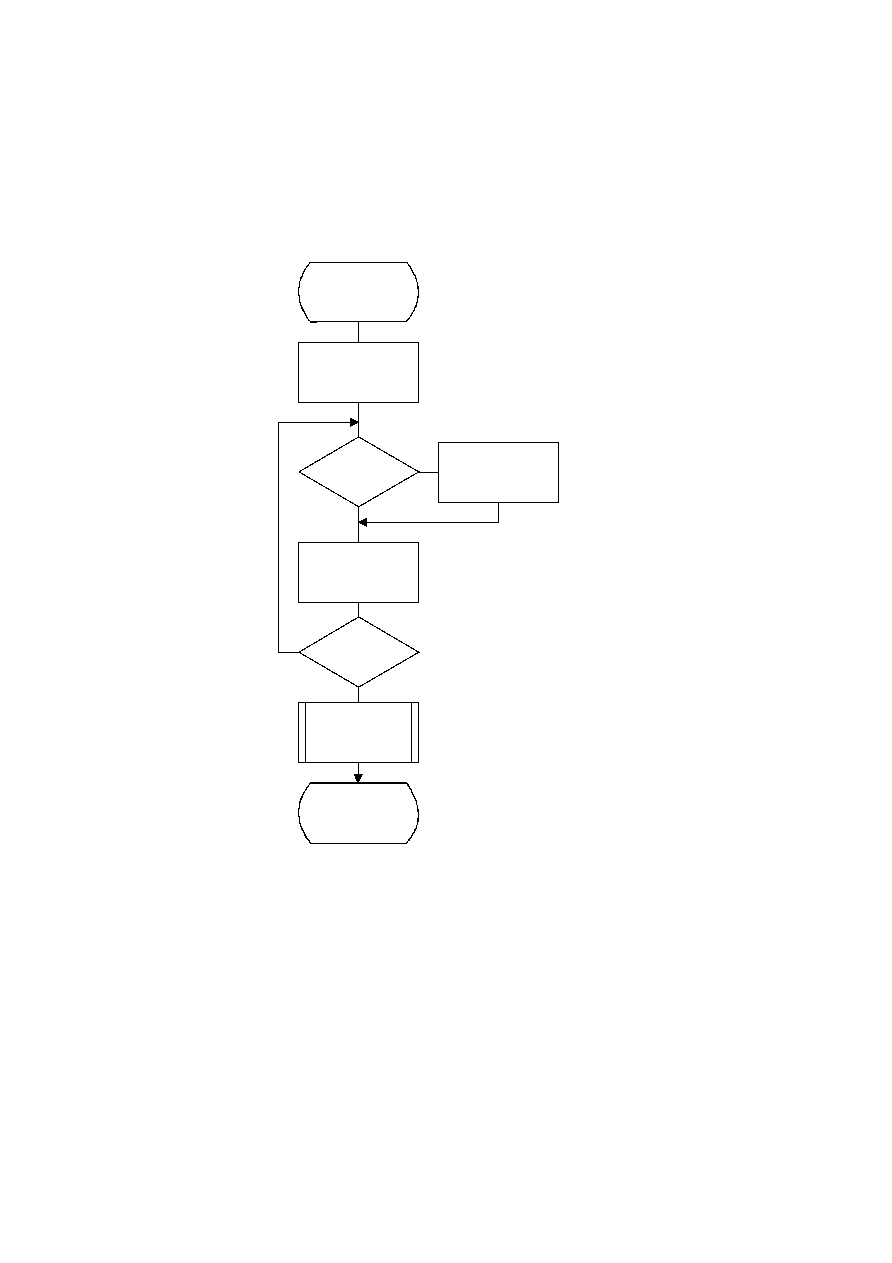ISO/IEC 10918-1 : 1993(E)
Once the code lengths for each symbol have been obtained, the number of codes of each length is obtained using the
procedure in Figure K.2. The count for each size is contained in the list, BITS. The counts in BITS are zero at the start of
the procedure. The procedure assumes that the probabilities are large enough that code lengths greater than 32 bits never
occur. Note that until the final Adjust_BITS procedure is complete, BITS may have more than the 16 entries required in
the table specification (see Annex C).
TISO1740-93/d112
Count_BITS
I = 0
I = I + 1
Done
Yes
No
Yes
No
I = 257
BITS(CODESIZE(I)) =
BITS(CODESIZE(I)) + 1
CODESIZE(I) = 0
?
Figure K.2 ­ Procedure to find the number of codes of each size
Figure K.2 [D112] = 16 cm = 625 %
146
CCITT Rec. T.81 (1992 E)